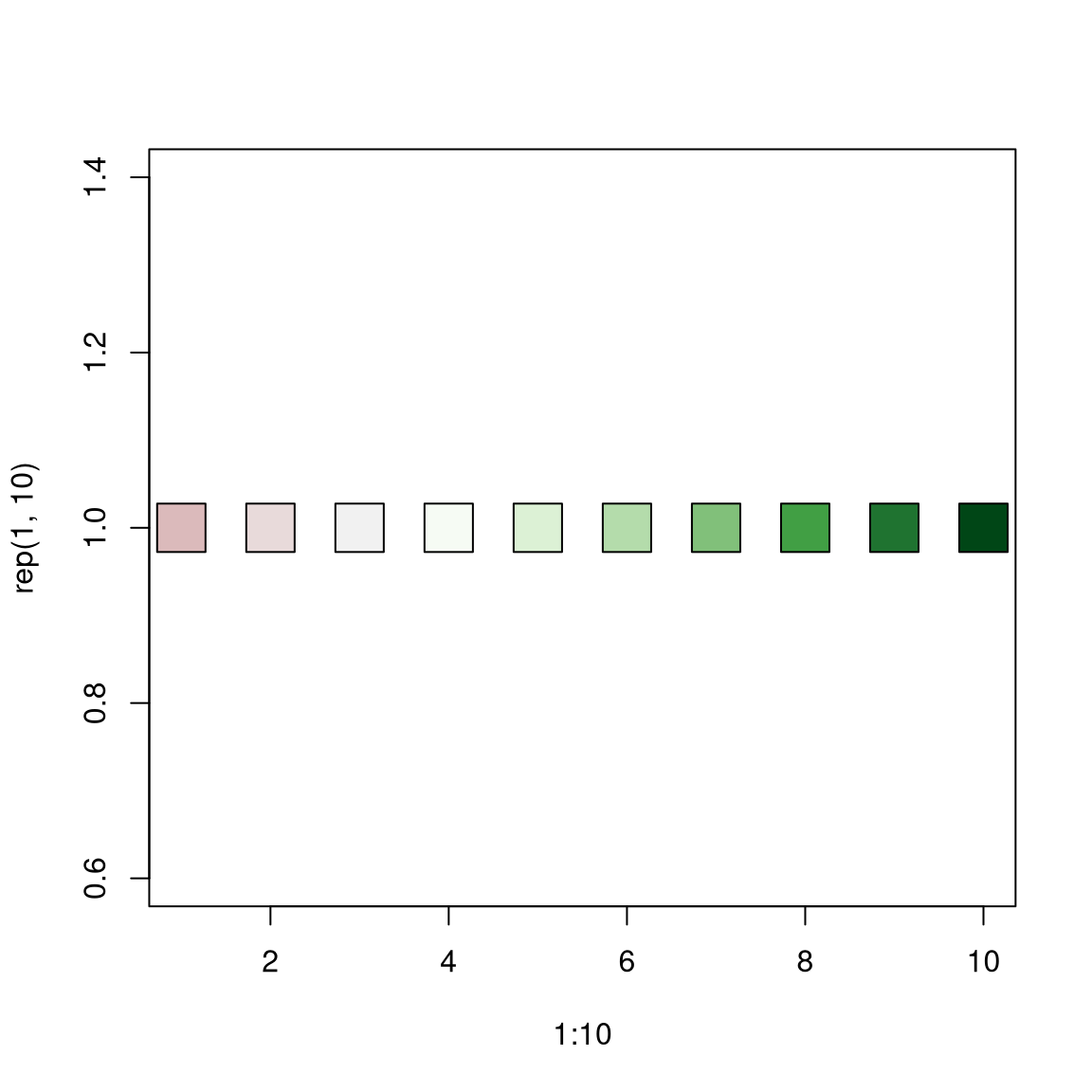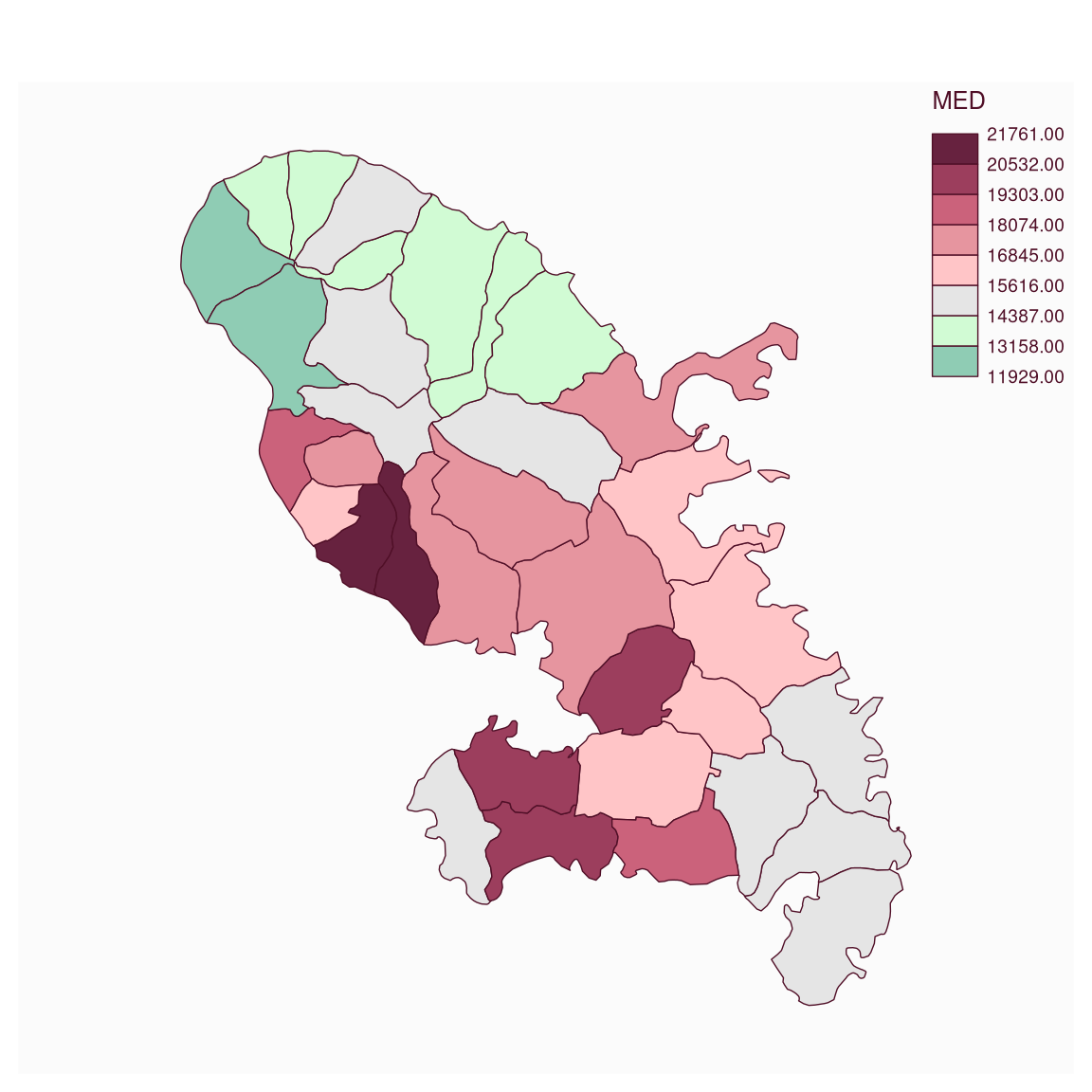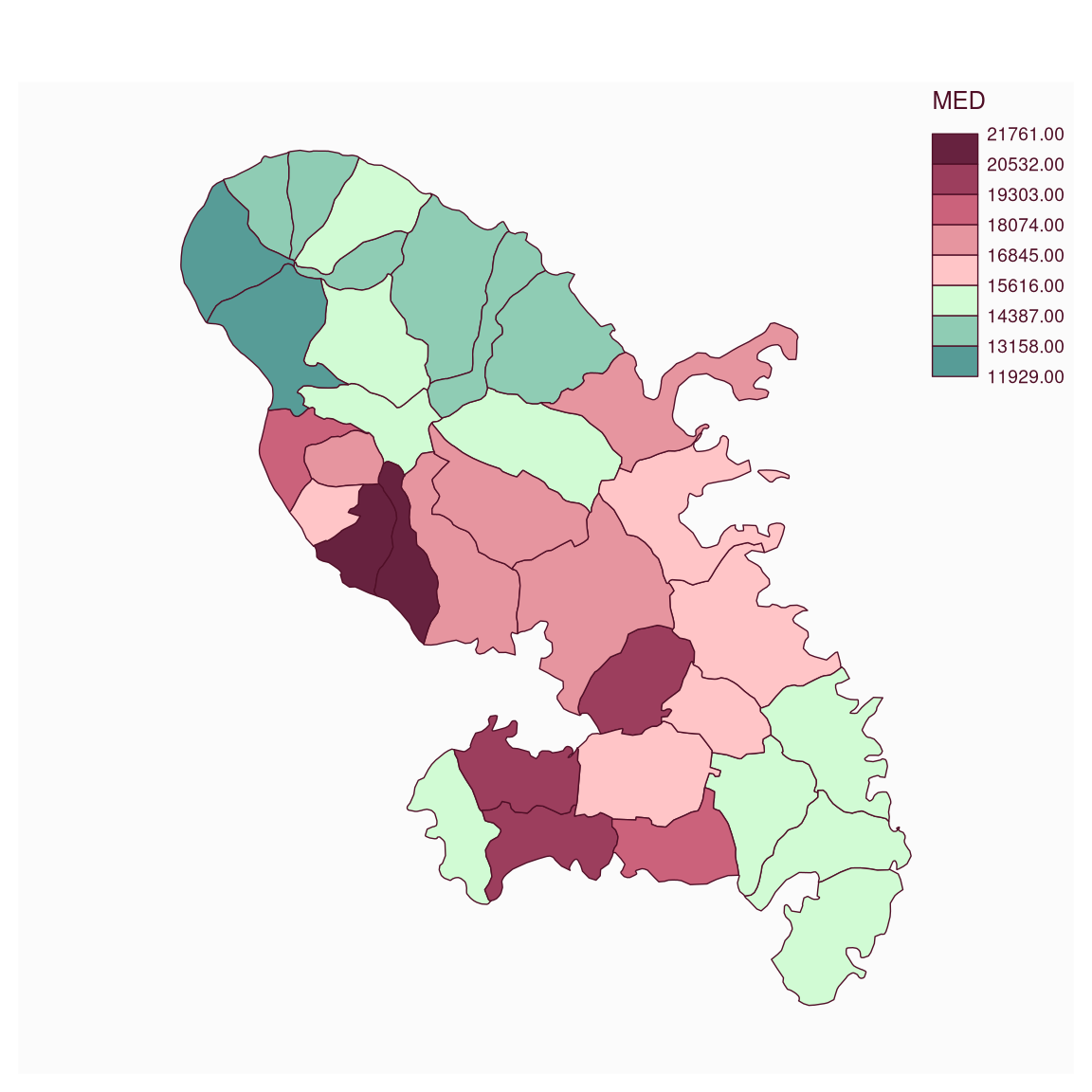mf_get_pal builds sequential, diverging and qualitative color palettes. Diverging color palettes can be dissymmetric (different number of colors in each of the two gradients).

mf_get_pal(n, palette, alpha = NULL, rev = c(FALSE, FALSE), neutral)

## Arguments

n

the number of colors (>= 1) to be in the palette.

palette

a valid palette name (one of hcl.pals()). The name is matched to the list of available palettes, ignoring upper vs. lower case, spaces, dashes, etc. in the matching.

alpha

an alpha-transparency level in the range [0,1] (0 means transparent and 1 means opaque), see argument alpha in hsv and hcl, respectively.

rev

logical indicating whether the ordering of the colors should be reversed.

neutral

a color, if two gradients are used, the 'neutral' color can be added between them.

## Value

A vector of colors.

## Details

See hcl.pals to get available palette names. If two gradients are used, the 'neutral' color can be added between them.

## Examples

cols <- mf_get_pal(n = 10, pal = "Reds 2")
plot(1:10, rep(1, 10), bg = cols, pch = 22, cex = 4)cols <- mf_get_pal(n = c(3, 7), pal = c("Reds 2", "Greens"))
plot(1:10, rep(1, 10), bg = cols, pch = 22, cex = 4)cols <- mf_get_pal(n = c(5, 5), pal = c("Reds 2", "Greens"))
plot(1:10, rep(1, 10), bg = cols, pch = 22, cex = 4)cols <- mf_get_pal(n = c(7, 3), pal = c("Reds 2", "Greens"))
plot(1:10, rep(1, 10), bg = cols, pch = 22, cex = 4)cols <- mf_get_pal(n = c(5, 5), pal = c("Reds 2", "Greens"), neutral = "grey")
plot(1:11, rep(1, 11), bg = cols, pch = 22, cex = 4)opar <- par(bg = "black")
cols <- mf_get_pal(n = c(7, 3), pal = c("Reds 2", "Greens"), alpha = c(.3, .7))
plot(1:10, rep(1, 10), bg = cols, pch = 22, cex = 4)par(opar)
cols <- mf_get_pal(
n = c(5, 5), pal = c("Reds 2", "Greens"),
rev = c(TRUE, TRUE)
)
plot(1:10, rep(1, 10), bg = cols, pch = 22, cex = 4)